# Java - Do While Loops

## Java - Do While Loops

• Repetitively executes set of statements once and then tests the given expression.
• Condition should result in Boolean type.
• The execution now continues if the condition is satisfied,else it is stopped.
• Hence there is a guarantee that the set of statements executes once for sure as indicated below: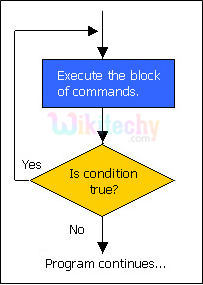Do while loop

#### Syntax :

```  do
{
\\ set of statement(s)
}
while (expression);```

#### Sample Code :

```public class JavaDoWhileLoop
{
public static void main(String[] args)
{
System.out.println("\n\nWikiTechy - Using Do While Loop\n");
int i = 10;
do
{
System.out.println("Value of i = " + i);
i+=2;
}
while(i <= 20);
}
}
```

#### Code Explanation :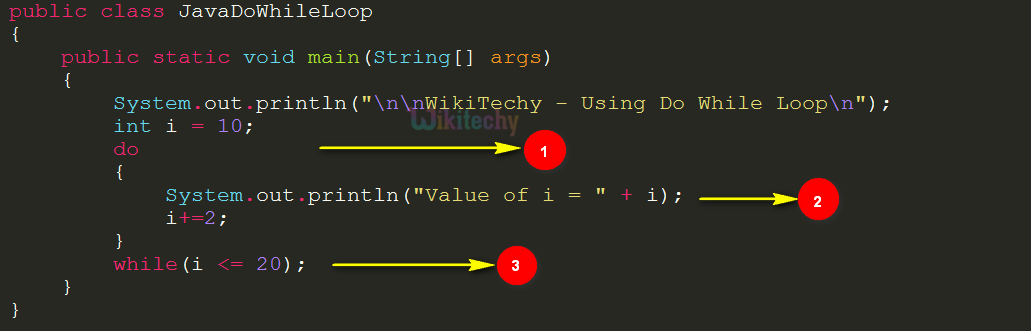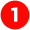The loop starts with the code “do”.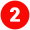The statements to print the value of i and increment i value by 2 are executed once before executing the while loop condition. Hence the code

```System.out.println("Value of i = " + i);
i+=2;```

executes and prints i value and increments it by 2.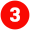Finally the while loop condition that is, while(i <= 20); is executed to decide on the code flow.

#### Output :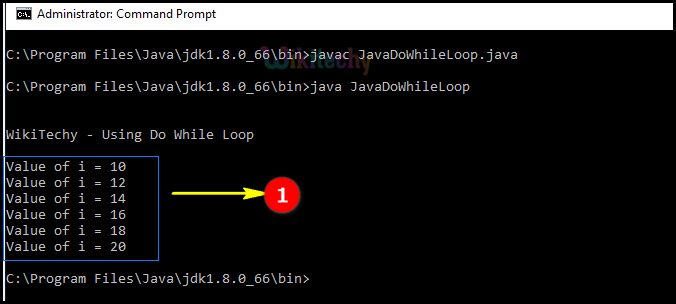Here in this java output, the “ i “ value has been incremented by 2 for each do while loop execution.

Bug Bounty
Webinar